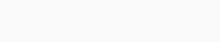# Solved – Scott’s and Freedman–Diaconis rules of the thumb for selecting bin width – disatvantages

Scott's and Freedman–Diaconis rules of the thumb are based on the following formula:In the article here it is stated that:

While these appear to be useful estimates for unimodal densities
similar to a Gaussian distribution, they are known to be
suboptimal for multimodal densities.

My question is, will Scott and Freedman–Diaconis rules of the thumb estimate the correct number of bins on distributions with more than one peak?

What are the disadvantages of these methods, and how to overcome them?

Contents

``set.seed(930) x = cbind(rnorm(50,12,2), rnorm(50,18,2), rnorm(50,25,2)) hist(x, prob=T, col="skyblue2"); rug(x) lines(density(x), col="red", lwd=2) ``